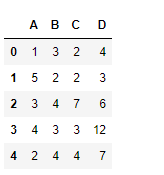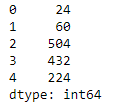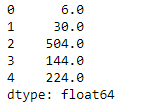Related Articles
Python | Pandas dataframe.product()
• Last Updated : 22 Nov, 2018

Python is a great language for doing data analysis, primarily because of the fantastic ecosystem of data-centric python packages. Pandas is one of those packages and makes importing and analyzing data much easier.

Pandas` dataframe.product()` function return the value of the product for the requested axis. It multiplies all the element together on the requested axis. By default the index axis is selected.

Syntax: DataFrame.product(axis=None, skipna=None, level=None, numeric_only=None, min_count=0, **kwargs)

Parameters :
axis : {index (0), columns (1)}
skipna : Exclude NA/null values when computing the result.
level : If the axis is a MultiIndex (hierarchical), count along a particular level, collapsing into a Series
numeric_only : Include only float, int, boolean columns. If None, will attempt to use everything, then use only numeric data. Not implemented for Series.
min_count : The required number of valid values to perform the operation. If fewer than min_count non-NA values are present the result will be NA.

Returns : prod : Series or DataFrame (if level specified)

Example #1: Use `product()` function to find product of all the elements over the column axis in the dataframe.

 `# importing pandas as pd``import` `pandas as pd`` ` `# Creating the dataframe ``df ``=` `pd.DataFrame({``"A"``:[``1``, ``5``, ``3``, ``4``, ``2``], ``                   ``"B"``:[``3``, ``2``, ``4``, ``3``, ``4``], ``                   ``"C"``:[``2``, ``2``, ``7``, ``3``, ``4``], ``                   ``"D"``:[``4``, ``3``, ``6``, ``12``, ``7``]})`` ` `# Print the dataframe``df`Let’s use the `dataframe.product()` function to find the product of each element in the dataframe over the column axis.

 `# find the product over the column axis``df.product(axis ``=` `1``)`

Output :Example #2: Use `product()` function to find the product of any axis in the dataframe. The dataframe contains `NaN` values.

 `# importing pandas as pd``import` `pandas as pd`` ` `# Creating the first dataframe ``df ``=` `pd.DataFrame({``"A"``:[``1``, ``5``, ``3``, ``4``, ``2``],``                   ``"B"``:[``3``, ``None``, ``4``, ``3``, ``4``], ``                   ``"C"``:[``2``, ``2``, ``7``, ``None``, ``4``],``                   ``"D"``:[``None``, ``3``, ``6``, ``12``, ``7``]})`` ` `# using prod() function to raise each element``# in df1 to the power of corresponding element in df2``df.product(axis ``=` `1``, skipna ``=` `True``)`

Output :Attention geek! Strengthen your foundations with the Python Programming Foundation Course and learn the basics.

To begin with, your interview preparations Enhance your Data Structures concepts with the Python DS Course. And to begin with your Machine Learning Journey, join the Machine Learning – Basic Level Course

My Personal Notes arrow_drop_up